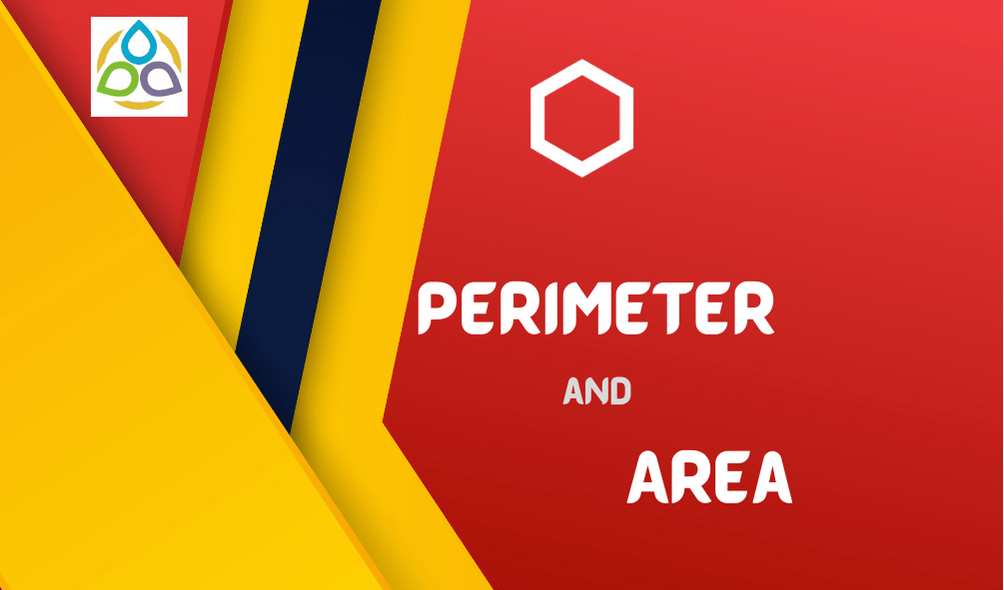# Perimeter and Area

Regular Questions

1.      Find the area of a square park whose perimeter is 320 m.

2.      Find the height x if the area of the parallelogram is 24 cm2 and the base is 4 cm.

3.      Find BC, if the area of the triangle ABC is 36 cm2 and the height AD is 3 cm.

4.         What is the circumference of a circle of diameter 10 cm (take π = 3.14)?

5.      What is the circumference of a circular disc of radius 14 cm (use π = 22/7)?

6.      Find the area of a circle of radius 30 cm (use π = 3.14).

7.      Diameter of a circular garden is 9.8 m. Find its area.

8.      Find the circumference of the circles with the following radius (take π = 22/7):

• (a) 14 cm
• (b) 28 mm
• (c) 21 cm

9.      Find the area of the following circles, given that (take π = 22/7):

• (a) radius = 14 mm
• (b) diameter = 49 m
• (c) radius = 5 cm.

10.    The radius of a circular pipe is 10 cm. What length of a tape is required to wrap once around the pipe (π = 3.14)?

11.    Sudha divides a circular disc of radius 7 cm in two equal parts. What is the perimeter of each semi-circular shape disc (use π = 22/7)?

1.Ty Hillesheim says:
2.zoritoler imol says: